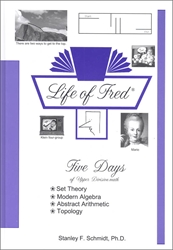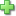Life of Fred: Five Days of Upper Division Math

Set Theory, Modern Algebra, Abstract Arithmetic, Topology

by Stanley F. Schmidt
Price: \$24.00

Life of Fred Five Days of Upper Division Math is the fourth book in the Life of Fred University Math series, meant to follow Linnear Algebra. This book acts as a sampler of advanced math for students who want to preview courses they want to take later. Featuring real math problems that give students a chance to solve puzzles rather than simply compute answers or learn procedures, this book give students an opportunity to create definitions, theorems, and proofs. Students will work through five days of Fred's adventures within four math subjects: set theory, modern algebra, abstract arithmetic, and topology. Each of the 139 assignments/puzzles/questions requires creativity rather than doing drill work and will take various lengths of time, from a minute to multiple hours.

Upper division (junior/senior) pure math is much different than calculus. No “word problems,” no formulas to memorize, no concrete applications—just puzzles to solve. Instead of learning procedures, students create definitions, theorems, and proofs.

These are the first five days of Fred’s teaching set theory, modern algebra, abstract arithmetic, and topology. Each of the 139 assignments/puzzles/questions that he gives his students calls for creativity rather than doing drill work. Some of these can be done in a minute. Some will take several hours to complete. They are all meant to be enjoyed. The goal is not to finish the book. It’s just like life.

1. The first day of set theory: cardinality of a set, set builder notation, naive set theory, modus ponens, seven possible reasons to give in a math proof, the high school geometry postulates are inconsistent, the proof that every triangle is isosceles, normal sets.
2. The first day of modern algebra: definition of a math theory, six properties of equality, formal definition of a binary operation, formal definition of a function, definition of a group, right cancellation law, left inverses, commutative law.
3. The first day of abstract arithmetic: circular definitions, unary operations, the successor function, natural numbers, the five Peano postulates, mathematical induction.
4. The first day of topology: topology is all about friendship, listed and counting subsets, open sets, the discrete topology, the three axioms of a topology, models for a topology, open intervals.
5. By the fifth day Fred will have covered the Schröder-Bernstein theorem (set theory), proved Lagrange’s theorem for subgroups of any group (modern algebra), defined the real numbers based only on the concept of “adding one” (abstract arithmetic), and explored continuous images of compact sets (topology).
Did you find this review helpful?
Related Categories
Recommended for...
Customer ReviewsClick here to write a review